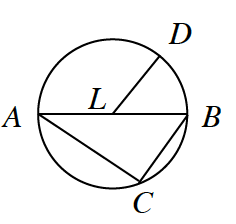### Home > CCG > Chapter 10 > Lesson 10.2.1 > Problem10-68

10-68.

In the diagram at right, $\overline{AB}$ is a diameter of $⊙L$. If $BC=5$ and $AC=12$ , use the relationships shown in the diagram to solve for the quantities listed below.1. $AB$

Start by finding the measure of $∠ACB$.

Use the Inscribed Angle Theorem, explained in the Math Notes box in Lesson 10.1.3, to determine the measure of $∠ACB$.

Since $ΔABC$ is a right triangle:
$(AC)^2 + (BC)^2 = (AB)^2$

$\begin{array}{l} 12^2 + 5^2 = \left(AB\right)^2 \\ 169 = \left(AB\right)^2 \\ AB = 13\ \text{units} \end{array}$

1. length of the radius of $⊙L$

Use the diameter, $\overline{AB}$, to find the radius.

1. $m∠ABC$

Use trigonometry to find the measures of the acute angles in $ΔABC$.

1. $m\overarc{ A C }$

$2 \cdot (m\angle ABC) = m \overarc{AC}$